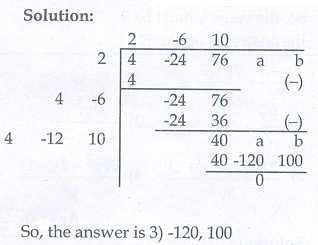# Chapter 3: Algebra - Online Test

Q1. A system of three linear equations in three variables is inconsistent if their planes
Explaination / Solution: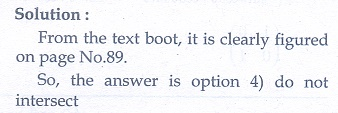Q2. The solution of the system x + y − 3x = −6 ,  − 7y + 7z = 7 , 3z = 9  is
Explaination / Solution: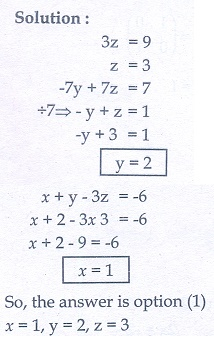Q3. If (x - 6) is the HCF of x2 - 2x - 24 and x2 - kx - 6 then the value of k is
Explaination / Solution: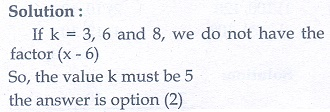Q4.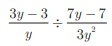is
Explaination / Solution:Q5.is not equal to
Explaination / Solution: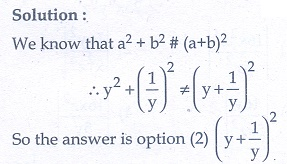Q6.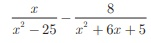gives
Explaination / Solution: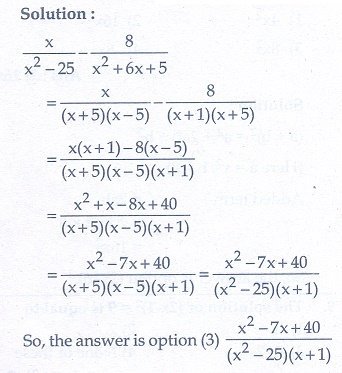Q7. The square root ofis equal to
Explaination / Solution:Q8. Which of the following should be added to make x4 + 64 a perfect square
Explaination / Solution: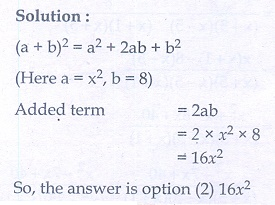Q9. The solution of  2x − 12  = 9 is equal to
Explaination / Solution: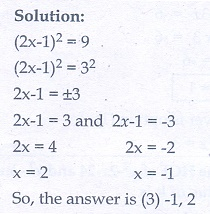Q10. The values of a and b if 4x 4 − 24x 3 + 76x 2 + ax +b is a perfect square are
Explaination / Solution: